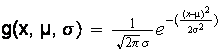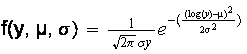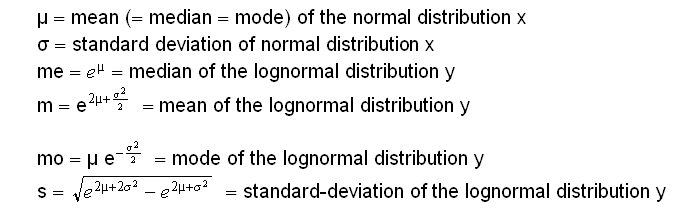# Cours avancé « Economie des inégalités » (Master APE, année M2)

Advanced course « Economics of Inequality » (Master APE, M2 year)

Thomas Piketty

Année universitaire 2008-2009

Course Notes B :

Pareto interpolation techniques for income and wealth distribution

1. The interpolation problem

Very often, available income or wealth distribution data takes of “grouped data”:

Income (or wealth or wage…) brackets : [s;s2],..., [s;si+1],..., [s;+µ]

Ni = number of individuals (or households or taxpayers…) between s and si+1

N* = total population

fi = Ni/N*   = proportion of population between s and si+1

Ni*= Ni+Ni+1+..+Np = total number of individuals above s

pi = Ni*/N*   = proportion of population above s

Yi  = total income of population between s and si+1

yi = Yi/Ni = average income of population between s and si+1

yi* = (Yi+...+Yp)/Ni* = average income of population above s

Sometime there is only information available on the Ni , not on the Yi

Even when micro data is available, sample size is very often insufficient, especially for the top of the distribution.

In both cases, one needs to make assumptions about the functional form of the distribution f(y) in order to use properly the available data = the interpolation problem

2. Lognormal distributions

y follows a lognormal distribution if and only if x = log(y) follows a normal distribution

I.e. if the density function g(x) can be written:The density function f(y) of the lognormal distribution can thus be written:Where:Conversely, one can express µ and σ as:(no closed form solution for distribution functions G(x)  and F(y), but available on all statistical software)

Exemple:

With m = 30 000€ and s = 20 000€, one gets me = 24 962€, mo = 17 281€, P90 = 54 297€

= fairly reasonable shape for the bottom 90% of the distribution

But P90-100 = 74 931€, P99 = 102 315€, P99-100 = 126 646€

= not reasonable for the top 10% of the distribution (and especially the top 1%)

>>> the problem of the lognormal distribution is that the upper tail is not fat enough

(i.e. the density function declines too fast toward 0)

3. Pareto distributions

The density and distribution functions f(y) and F(y) of a Pareto distribution y can be written as follows :

G(y) = 1-F(y) = (k/y)a   (k>0, a>1)

f(y)=aka/y(1+a)

Key property n°1 : ratio average/threshold = constant

Note y*(y) the average income (or wealth, or wage) of the population above threshold y. Then y*(y) can be expressed as follows :

y*(y) = [ òz>y z f(z)dz ] / [ òz>y f(z)dz ] = [ òz>y dz/za ] / [ òz>y dz/z(1+a) ] = ay/(a-1)

I.e.

y*(y)/y = b = a/(a-1)

(and a = b/(b-1) )

In practice :

For top incomes:

France today: b = 1.7-1.8 (a=2.2-2.3)

France interwar, US interwar, US today:  b = 2.2-2.3 (a=1.7-1.8)

For top wealth:

France today: b = 2.2-2.3 (or 2-2.5)

Higher b coefficients = fatter upper-tail = higher concentration

Key property n°2 : log-linearity

If one plots log(1-F(y)) against log(y), one gets a straight line with slope = -a

log(1-F(y)) = log(k) – a log(y)

Note : if one plots log(f(y)) against log(y), one gets a straight line with slope -(1+a) : og(f(y)) = log(aka) – (1+a) log(y)

Intuitive meaning of coefficient a: if one raises y by 1%, by how many % does the proportion above y declines? In practice this coefficient rises from the bottom of the distribution (where it is usually much below 1) to the middle top of the distribution; the point is that is tends to stabilize around 1,8-2,2 around a large plateau P90-99,99 (= why Pareto needs to complement lognormal: with full lognormal coefficient a rises continuously, and b declines continuously to very low levels), before of course going to infinity (b goes to 1) for the very last top incomes

4. Estimating Pareto parameters from grouped data

Grouped data :

Income (or wealth or wage…) brackets : [s;s2],..., [s;si+1],..., [s;+µ]

Ni = number of individuals (or households or taxpayers…) between s and si+1

N* = total population

fi = Ni/N*   = proportion of population between s and si+1

Ni*= Ni+Ni+1+..+Np = total number of individuals above s

pi = Ni*/N*   = proportion of population above s

Yi  = total income of population between s and si+1

yi = Yi/Ni = average income of population between s and si+1

yi* = (Yi+...+Yp)/Ni* = average income of population above s

Sometime there is only information available on the Ni , not on the Yi : then we say that all we have is “frequency information”

Otherwise we say we have both frequency and income information

Methodology M1: Average income interpolation methodology

(Piketty 2001, 2003, Piketty-Sez 2003)

This methodology uses both frequency and income information, and relies on property 1.

In order to estimate P99,5 (say), pick (si,pi) and (si+1,pi+1) such that pi+1 < 0,5% < pi), and compute b and k using the following formulas :

b = yi* / si

a = b / (b-1)

k = sipi1/a

Then use these coefficients to estimate P99,5=k/(0,0051/a) and P99,5-100=(a/(a-1))P99,5

Methodology M2 : Standard log-linear interpolation methodology

(Pareto (1896), Kuznets (1953), Feenberg et Poterba (1993))

This methodology uses solely frequency information, and relies on property 2.

In order to estimate P99,5 (say), pick (si,pi) and (si+1,pi+1) such that pi+1 < 0,5% < pi), and compute a and k using the following formulas :

a = log(pi/pi+1) / log(si+1/si)

b = a / (a-1)

k = sipi1/a

Then use these coefficients to estimate P99,5=k/(0,0051/a) and P99,5-100=(a/(a-1))P99,5

Which of these three methodologies should be used?

If one has both frequency and income information, then it is better to use methodology M2

If one has solely

There also exists more complicated methodologies taking explicitely into account the fact that Pareto parameters are not constant (see e.g. Cowell 2000, Zucman 2008)

Some references on interpolation techniques

Atkinson (2007), chapter 2 in Atkinson-Piketty 2007

D. G. Champernowne and F. Cowell, “Economic Inequality and Income Distribution”, Cambridge University Press 1998

Cowell, Measuring Inequalities, electronic manuscript 2000

Cowell-Mehta, “The estimation and interpolation of inequality measures”, Review of Economic Studies 1982, vol.49 pp.273-290

Piketty (2001, Appendix B)